FREE SHIPPING ON ALL U.S. ORDERS AND 30 DAY RETURNS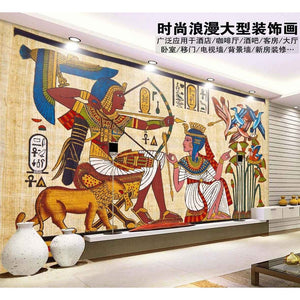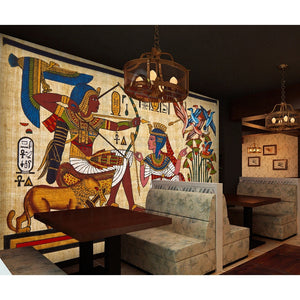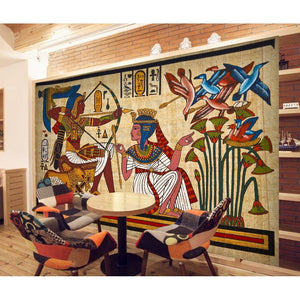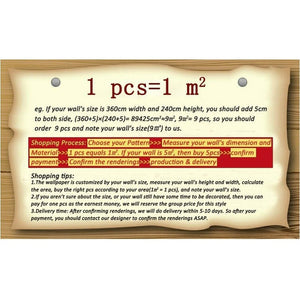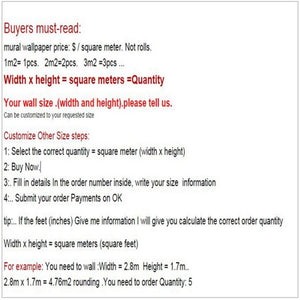# Ancient Egyptian murals Dream living room bedroom TV background wall paper 3D mural wallpaper

Regular price \$76.00 \$76.00 Sale

Wallpaper non standard sizes
According to your wall size customization
(1 piec Price = 1 square meter)
1 square meter = 10.8 square feet

(Width × height = square meters)
Width multiplied by height equal to the amount you need to buy
For example
My wall measurement high 2M width 3M (2 × 3 = 6 square meters) buy 6

(More than 0.5 square meters need to buy one square meter Less than 0.5 square meters free)

•Note:
1:The wallpaper is customized according to your size (Height and Width), if you are intersted in any of the items, please leave us message or send e-mail, which includes the wallpaper height and width you are going to order。

2:Please share with your length x height of wall, we will cut this wallpaper according to your length x height.
For example:
If you buy 1 square meter(10.764 square feet):Length x Height is equal to 10.764 square feet.
If you buy 2 square meter(21.528 square feet):Length x Height is equal to 21.528 square feet.
And so on.......

3:No message for the size information ,we will send our default size as follow

Quantity: 1 square meter = 125cm (W) x 80cm (H) (4'1 "x 2'6") ft
Quantity: 2 square meter = 200cm (W) x 100cm (H) (6'5 "x 3'2") ft
Quantity: 3 square meter = 208cm (W) x 146cm (H) (6'8 "x 4'8") ft
Quantity: 4 square meter = 200cm (W) x 200cm (H) (6'5 '' x 6'5 '') ft
Quantity: 5 square meter = 250cm (W) x 200cm (H) (7'6 '' x6'5 '') ft
Quantity: 6 square meter = 300cm (W) x 200cm (H) (9'8 '' x 6'5 '') ft
Quantity: 7 square meter = 300cm (W) x 250cm (H) (9'8 "x 8'2") ft
Quantity: 8 square meter = 400cm (W) x 200cm (H) (13'6 "x 6'5") ft
Quantity: 9 square meter = 350cm (W) x 260cm (H) (11'4 "x 8'7") ft
Quantity: 10 square meter = 360cm (W) x 280cm (H) (11'8 "x 9'2") ft
Quantity: 12 square meter = 400cm (W) x 300cm (H) (13'6 "x 9'8") ft
Quantity: 16 square meter = 500cm (W) x 320cm (H) (16'4 "x 10'5") ft